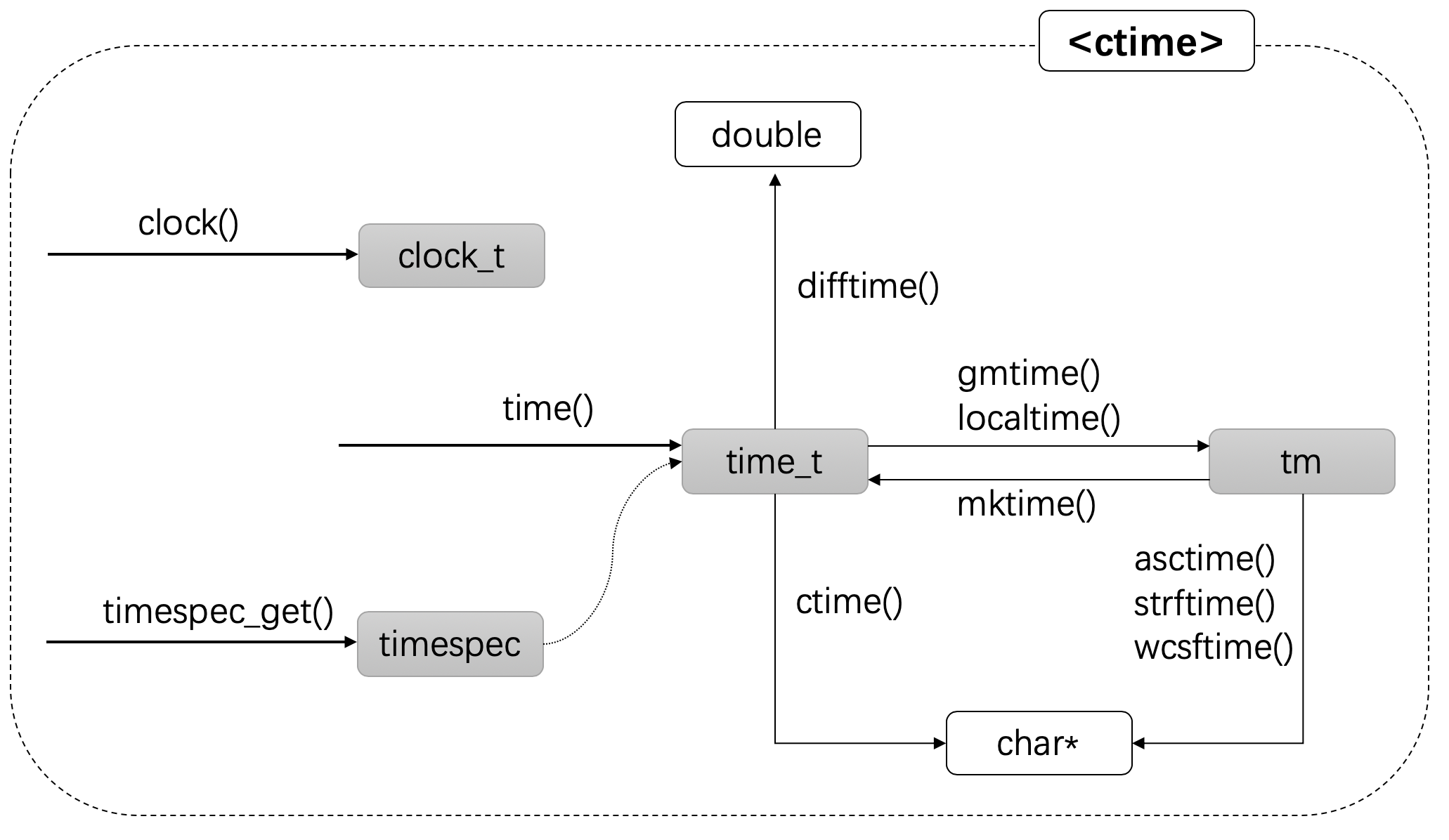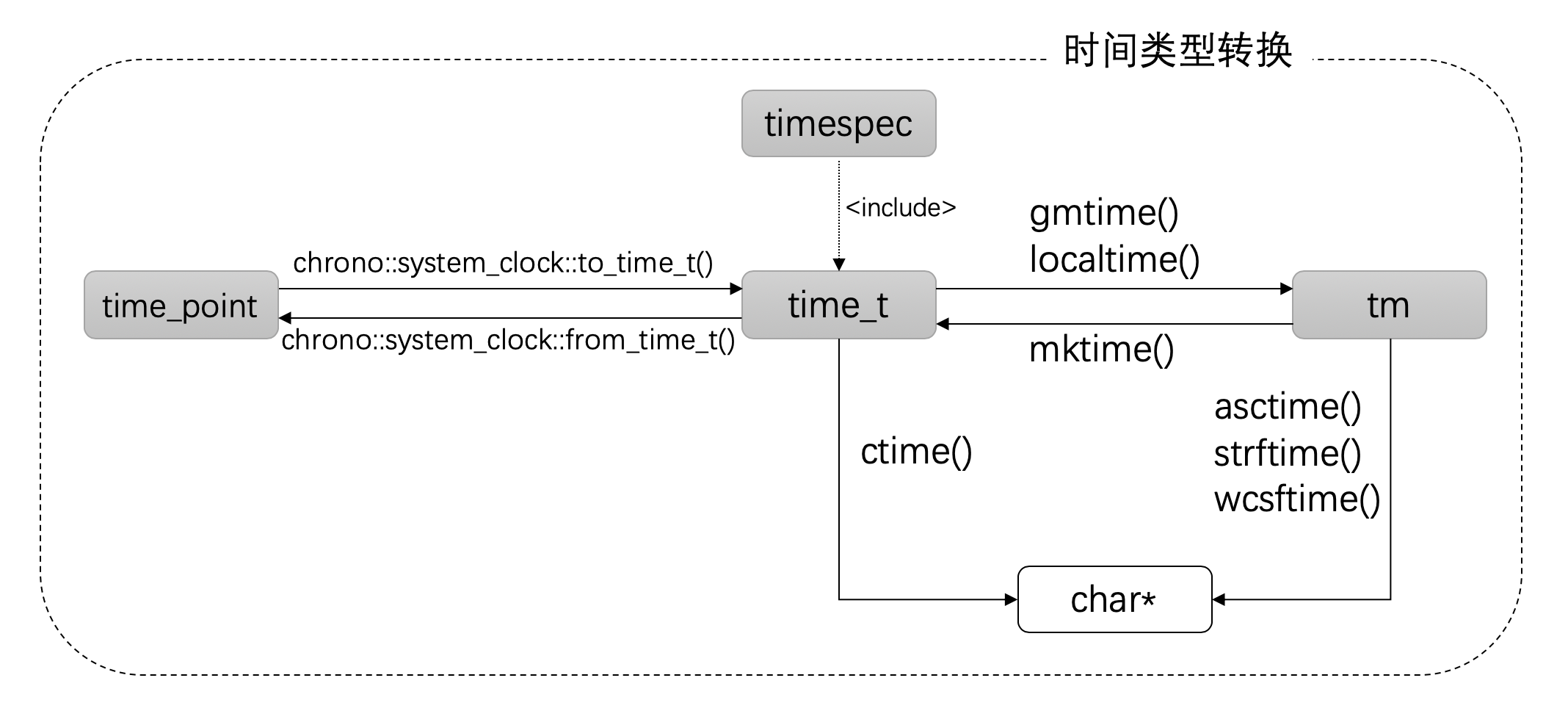## C++ 日期和时间编程

Posted on Feb 3, 2020# 介绍

C++中可以使用的日期时间API主要分为两类：

• C-style 日期时间库，位于<ctime>头文件中。这是原先<time.h>头文件的C++版本。
• chrono库：C++ 11中新增API，增加了时间点，时长和时钟等相关接口。

# 代码示例

git clone https://github.com/paulQuei/cpp-date-time.git


#include <chrono>
#include <ctime>
#include <iostream>

using namespace std;


# C-style 日期时间库

C-style 日期时间库中包含的函数和数据类型说明如下：

## 函数

std::clock_t clock() 返回自程序启动时起的处理器时钟时间
std::time_t time(std::time_t* arg) 返回自纪元起计的系统当前时间
double difftime(std::time_t time_end,
std::time_t time_beg)

int timespec_get(std::timespec* ts, int base)$^{*}$ 返回基于给定时间基底的日历时间
char* ctime(const std::time_t* time) 转换 time_t 对象为文本表示
char* asctime(const std::tm* time_ptr) 转换 tm 对象为文本表示
std::size_t strftime(char* str,
std::size_t count, const char* format,
const std::tm* time)

std::size_t wcsftime( wchar_t* str,
std::size_t count, const wchar_t* format,
const std::tm* time)

std::tm* gmtime(const std::time_t* time) 将time_t转换成UTC表示的时间
std::tm* localtime(const std::time_t *time) 将time_t转换成本地时间
std::time_t mktime(std::tm* time) 将tm格式的时间转换成time_t表示的时间

## 数据类型

time_t 从纪元起的时间类型
tm 日历时间类型
timespec$^{*}$ 以秒和纳秒表示的时间
clock_t 进程运行时间
size_t sizeof 运算符返回的无符号整数类型

## 结构梳理• clock函数是相对独立的一个函数，它返回进程运行的时间，具体描述见下文。
• time_t描述了纪元时间，通过time函数可以获得它。但它只能精确到秒级别。
• timespec类型在time_t的基础上，增加了纳秒的精度，通过timespec_get获取。这是C++17上新增的
• tm是日历类型，因为它其中包含了年月日等信息。通过gmtime，localtime和mktime函数可以将time_t和tm类型互相转换。
• 考虑到时区的差异，因此存在gmtime和localtime两个函数。
• 无论是time_t还是tm结构，都可以将其以字符串格式输出。ctime和asctime输出的格式是固定的。如果需要自定义格式，需要使用strftime或者wcsftime函数。

## 进程运行时间

clock函数返回进程迄今为止所用的处理器时间。单独调度该函数一次所返回的值是没有意义的，只有两次不同值的差才有意义。

clock_t time1 = clock();
double sum = 0;
for(int i = 0; i < 100000000; i++) {
sum += sqrt(i);
}
clock_t time2 = clock();

double t = ((double)(time2 - time1)) / CLOCKS_PER_SEC ;
cout << "CLOCKS_PER_SEC: " << CLOCKS_PER_SEC << endl;
cout << "Process running time: " << t << "s" << endl;


CLOCKS_PER_SEC: 1000000
Process running time: 0.80067s


## 关于纪元时间

time_t epoch_time = time(nullptr);
cout << "Epoch time: " << epoch_time << endl;


Epoch time: 1577433897


time函数接受一个指针，指向要存储时间的对象，通常可以传递一个空指针，然后通过返回值来接受结果。

## 计算时间差

time_t time1 = time(nullptr);
double sum = 0;
for(int i = 0; i < 1000000000; i++) {
sum += sqrt(i);
}
time_t time2 = time(nullptr);

double time_diff = difftime(time2, time1);
cout << "time1: " << time1 << endl;
cout << "time2: " << time2 << endl;
cout << "time_diff: " << time_diff << "s" << endl;


time1: 1577434406
time2: 1577434414
time_diff: 8s


## 输出时间和日期

time_t now = time(nullptr);
cout << "Now is: " << ctime(&now);


Now is: Fri Dec 27 16:17:45 2019


## UTC时间与本地时间

struct tm {
int tm_sec;
int tm_min
int tm_hour;
int tm_mday;
int tm_mon;
int tm_year;
int tm_wday;
int tm_yday;
int tm_isdst;
};


• tm_mon 并非我们人类理解的月份，而是[0, 11]的范围。因此在某些时候你可能要对其+1.
• tm_year 是自1900起之年，因此你可能也需要对其 +1900。

asctime可以直接将tm结构转换成人类理解的字符串格式，不过其格式是固定的：Www Mmm dd hh:mm:ss yyyy\n。对于有特定格式要求，可以使用下文提到的其他方法。

time_t now = time(nullptr);

tm* gm_time = gmtime(&now);
tm* local_time = localtime(&now);

cout << "gmtime: " << asctime(gm_time);
cout << "local_time: " << asctime(local_time);


gmtime: Fri Dec 27 08:36:14 2019
local_time: Fri Dec 27 16:36:14 2019


## 自定义时间格式

time_t now = time(nullptr);
tm* t = localtime(&now);

cout << "Now is: " << t->tm_year + 1900 << "/" << t->tm_mon + 1<< "/" << t->tm_mday << " ";
cout << t->tm_hour << ":" << t->tm_min << ":" << t->tm_sec << endl;


char buffer;
strftime(buffer, 32, "%Y/%m/%d %H:%M:%S", t);
cout << "Now is: " << buffer << endl;


Now is: 2019/12/27 16:40:39


## 纳秒精度的timespec

$1 秒(Second) = 10^{3} 毫秒(millisecond)= 10^{6} 微妙(microsecond) = 10^{9}纳秒(nanosecond)$

timespec类型结构如下：

struct timespec {
std::time_t tv_sec;
long tv_nsec;
};


timespec ts;
timespec_get(&ts, TIME_UTC);
char buff;
strftime(buff, sizeof buff, "%D %T", std::gmtime(&ts.tv_sec));
printf("Current time: %s.%09ld UTC\n", buff, ts.tv_nsec);


timespec_get的第二个参数是一个整形表达的base。目前标准只定义了TIME_UTC。

Current time: 12/27/19 09:03:29.497456000 UTC


# chrono 库

“chrono”是英文chronology的缩写，其含义是“年表；年代学”。

## 时钟

system_clock 系统时钟
high_resolution_clock 拥有可用的最短嘀嗒周期的时钟

system_clock 的时间来源是系统时钟，而系统时间随时都可能被调整。所以如果你需要计算两个时间点的时间差，这不是一个好的选择。因为如果两次时间差中间系统时间被调整了，其结果就没有意义了。

high_resolution_clock 表示实现提供的拥有最小计次周期的时钟。它可以是 system_clock 或 steady_clock 的别名，也可能是第三个独立时钟。

$now()$ 静态成员函数，返回当前时间，类型为clock::time_point
time_point 成员类型，当前时钟的时间点类型，见下文“时间点”
duration 成员类型，时钟的时长类型，见下文“时长”
rep 成员类型，时钟的tick类型，等同于clock::duration::rep
period 成员类型，时钟的单位，等同于clock::duration::period

std::chrono::time_point<std::chrono::steady_clock> now = std::chrono::steady_clock::now();



### 与C-style转换

system_clock与另外两个clock不一样的地方在于，它还提供了两个静态函数用来与std::time_t来回转换：

to_time_t 转换系统时钟时间点为 std::time_t
from_time_t 转换 std::time_t 到系统时钟时间点auto now = chrono::system_clock::now();
time_t time = chrono::system_clock::to_time_t(now);
cout << "Now is: " << ctime(&time) << endl;


## 时长

### ratio

std::ratio描述了编译时的有理数，这是一个模板类。有了这个类型之后，我们就可以表示任意精度的值了。

std::ratio<1, 1000>       milliseconds;
std::ratio<1, 1000000>    microseconds;
std::ratio<1, 1000000000> nanoseconds;


ratio_add<ratio<5, 7>, ratio<59, 1023>> result;
double value = ((double) result.num) / result.den;
cout << result.num << "/" << result.den << " = " << value << endl;


5528/7161 = 0.771959


### 时长类型

std::chrono::nanoseconds duration</*至少 64 位的有符号整数类型*/, std::nano>
std::chrono::microseconds duration</*至少 55 位的有符号整数类型*/, std::micro>
std::chrono::milliseconds duration</*至少 45 位的有符号整数类型*/, std::milli>
std::chrono::seconds duration</*至少 35 位的有符号整数类型*/>
std::chrono::minutes duration</*至少 29 位的有符号整数类型*/, std::ratio<60»
std::chrono::hours duration</*至少 23 位的有符号整数类型*/, std::ratio<3600»

duration类的count()成员函数返回具体数值。

### 时长运算

duration_cast 进行时长的转换
floor（C++17） 以向下取整的方式，将一个时长转换为另一个时长
ceil（C++17） 以向上取整的方式，将一个时长转换为另一个时长
round（C++17） 转换时长到另一个时长，就近取整，偶数优先
abs（C++17） 获取时长的绝对值

chrono::hours two_hours(2);
chrono::minutes five_minutes(5);

auto duration = two_hours + five_minutes;
auto seconds = chrono::duration_cast<chrono::seconds>(duration);
cout << "02:05 is " << seconds.count() << " seconds" << endl;


02:05 is 7500 seconds


• h表示小时
• min表示分钟
• s表示秒
• ms表示毫秒
• us表示微妙
• ns表示纳秒

using namespace std::chrono_literals;
auto two_hours = 2h;
auto five_minutes = 5min;


## 时间点

### 时间点运算

时间点 + 时长 = 时间点

时间点 - 时间点 = 时长


auto start = chrono::steady_clock::now();
double sum = 0;
for(int i = 0; i < 100000000; i++) {
sum += sqrt(i);
}

auto time_diff = end - start;
auto duration = chrono::duration_cast<chrono::milliseconds>(time_diff);
cout << "Operation cost : " << duration.count() << "ms" << endl;


# 展望 C++ 20

• 更多类型的时钟，例如：utc_clock，tai_lock，gps_clock等。
• 时钟以及时间点的转换
• Calendar支持
• 时区支持

Contents